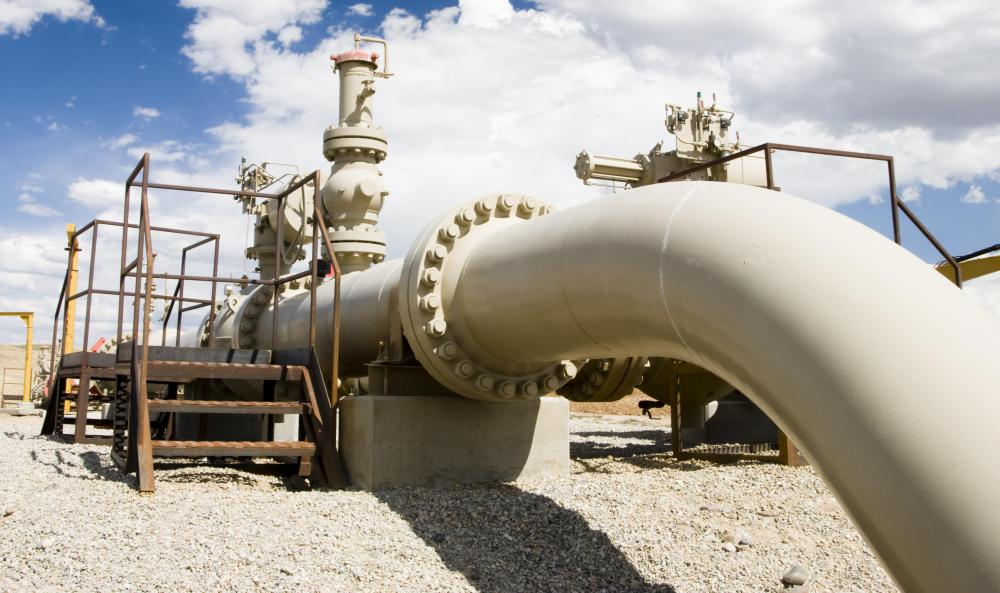# What Is a Venturi Meter?

Larry Ray Palmer

A venturi meter, or venturi flow meter, is a device used to measure the velocity, or flow rate, of fluid flowing through a pipeline. The venturi meter constricts the flow using a Herschel venturi tube. As the liquid flows through the pipeline, the device measures the pressure of the liquid before it enters the venturi tube and as it exits the constricted area. These measurements are then compared to figure the volumetric flow rate of the fluid. The flow meter is commonly used in plumbing applications to determine the flow of fluids such as water, liquid propane, and oil.A venturi meter is a device used to measure the flow rate of fluid flowing through a pipeline.

For this procedure, the venturi tube is typically a long pipe with conically shaped entry and exit points. The entry point is usually a thirty degree cone which constricts to a five degree cone at the exit. The venturi meter results in less degradation of the head pressure due to the tube's design.

The venturi meter can accurately measure flow rate using Bernoulli's principle. Bernoulli stated that the velocity of liquid increases in direct proportion to a decrease in pressure. When the liquid is forced through a constricted pipeline, it begins to move at a higher rate of speed because the majority of the pressure is held behind the constriction.

A common example of Bernoulli's principle in action can be found in the nozzle of a garden hose. As the nozzle is turned fully open, the water flows slowly and usually falls just a short distance from the nozzle. When the nozzle is turned towards the closed position, it restricts the flow of the water and increases its velocity. The pressure held behind the nozzle builds and the velocity can carry the water for great distances.

The venturi meter precisely calculates the volumetric flow rate of a fluid using a complex formula. This computation considers the radius of the pipeline, whether or not the measured liquid is compressible, and the total volume of fluid present. Various coefficients are added to compensate for the viscosity of the fluid, changes in the conical angles of the venturi, and other variables. When these numbers are calculated, along with the pressure measurements taken by the venturi meter, an accurate description of the liquid's flow rate can be ascertained.

## You might also Like